# MATH 251: Calculus 3, SET8

## 12: Vectors and the Geometry of Space

### 12.5: Equations of Lines and Planes

These problems are done with the CAS. See Hand Solutions for details.

#### 1. [831/4]

A line through a given point parallel to a specified line is determined.
syms t x y z
P = sym([0,14,-10]), v = sym([2,-3,9])
P =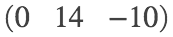v =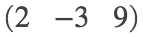L = P + t*v % vector equation
L =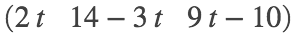parametric_eqs = [x y z] == L
parametric_eqs =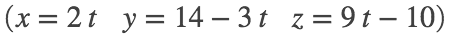#### 2. [831/10]

A line through a given point perpendicular to a pair of vectors is determined.
Vector and parametric equations for the line are given.
Symmetric equations are.
syms t x y z
P = sym([2,1,0]), u = sym([1 1 0]), v = sym([0,1 1])
P =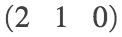u =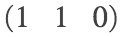v =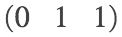c = cross(u,v)
c =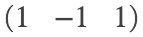L = P + c*t % vector equation
L =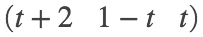parametric_eqs = [x y z] == L
parametric_eqs =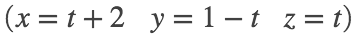symmetric_eqs =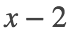#### 3. [831/18]

Parametric equations for the directed line segment between two points are determined.
Note that the domain of the parameter t is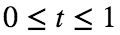since the line segment is of finite extent.
syms t x y z
A = sym([-2,18,31]), B = sym([11,-4,48])
A =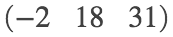B =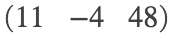v = B-A % direction vector from A to B
v =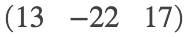L = A + t*v
L =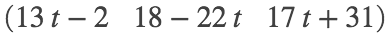parametric_eqs = [x y z] == L
parametric_eqs =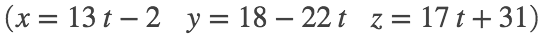#### 4. [831/20]

Given vector equations for two lines in space, we determine if they are parallel, intersecting, or skew (neither).
A direction vector for a line may be obtained by rewriting the vector equation as P + t v and reading off v.
(More simply, as we'll learn in Section 13.2, just take the derivative of the vector function w.r.t. the parameter.)
syms s t
L1 = [5-12*t 3+9*t 1-3*t]
L1 =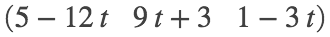L2 = [3+8*s -6*s 7+2*s]
L2 =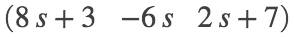v = diff(L1,t), w = diff(L2,s)
v =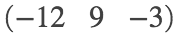w =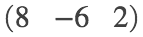m = v./w
m =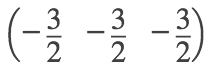% Since v = -3/2 * w, the direction vector v is a constant multiple of w.
% Accordingly, the direction vectors and hence the lines are parallel.

#### 5. [832/34]

An equation of the plane through three points is determined once a norm vector perpendicular to the plane is found.
syms x y z
A = sym([3 0 -1]), B = sym([-2 -2 3]), C = sym([7 1 -4])
A =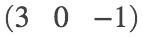B =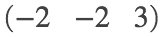C =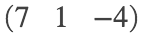AB = B-A, AC = C-A, n = cross(AB,AC)
AB =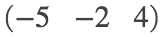AC =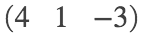n =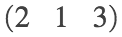eq_of_plane = dot(n,[x y z]) == dot(n,A)
eq_of_plane =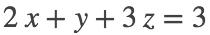f = solve(eq_of_plane, z)
f =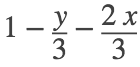% An illustrative plot is provided.
figure
fsurf(f, 'r', 'MeshDensity', 7)
xlabel('x'); ylabel('y'); zlabel('z')
axis equal
title('SET8, 832/34')
view(-13,55)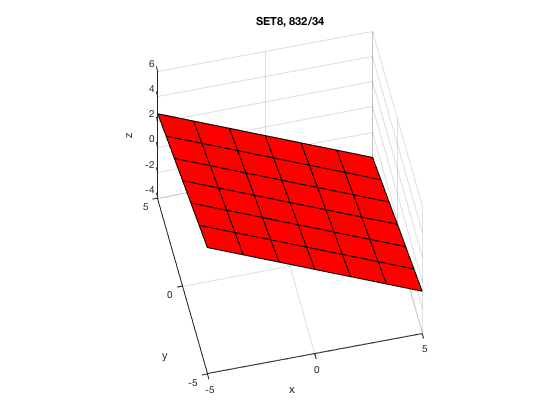#### 6. [832/46]

We determine the point were a line intersects a plane.
syms t x y z
L = [t-1 1+2*t 3-t]
L =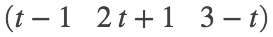plane = 3*x - y + 2*z == 5
plane =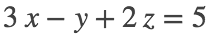eq = subs(plane, [x y z], L)
eq =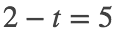ti = solve(eq, t)
ti =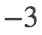P = subs(L, t, ti) % point of intersection
P =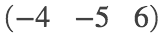% Here's a plot illustrating the situation.
f = solve(plane, z)
f =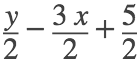figure
fsurf(f, [-7 -1 -8 -2], 'g', 'MeshDensity', 7); hold on
fplot3(L(1), L(2), L(3), [-9 3], 'm', 'LineWidth', 3)
plot3(-4, -5, 6, 'bo', 'MarkerFaceColor', 'b', 'MarkerSize', 14)
xlabel('x'); ylabel('y'); zlabel('z')
alpha 0.4
axis equal; axis([-7 -1 -8 -2 3 9])
title('SET8, 832/46')
view(-43,7)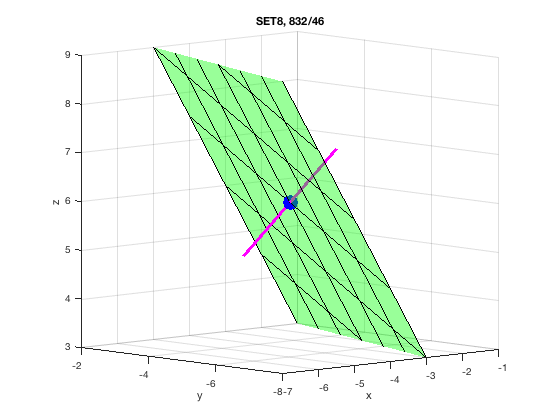#### 7. [832/62]

The set of points equidistant from two points in space is a plane perpendicular to the line segment connecting the points and containing the midpoint of said line segment. (It is the spacial analog of a perpendicular bisector through the midpoint of a line segment in a plane.)
syms x y z
A = sym([2 5 5]), B = sym([-6 3 1])
A =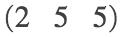B =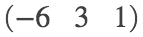M = (A + B)/2 % midpoint of line segment
M =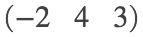n = A-B % a normal vector to the plane
n =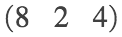plane = dot(n,[x y z]) == dot(n,M)
plane =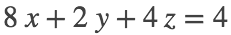more_simply = plane/2
more_simply =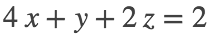#### 8. [832/70]

The distance between a point and a line in space is computed using the formula given in Exercise 45 of Section 12.4
(We check our answer as Calculus 1 optimization problem.)
syms t
P = sym([0 1 3])
P =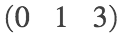L(t) = [2*t 6-2*t 3+t]
L(t) =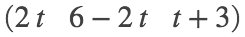Q = L(0), R = L(1)
Q =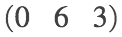R =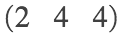a = R-Q, b = P-Q
a =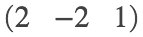b =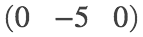n = cross(a,b)
n =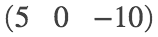d = simplify(norm(n) / norm(a))
d =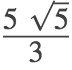d_appx = double(d) % in cm
d_appx = 3.7268
% CHECK: Equivalently, we'll minimize the
% square of the distance from P to a point on L.
g = L(t) - P
g =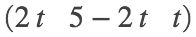f(t) = expand(sum(g.^2)) % square of distance
f(t) =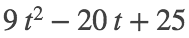Df(t) = diff(f(t), t) % Take derivative...
Df(t) =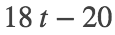tm = solve(Df(t)==0, t) % ...set it to zero and solve.
tm =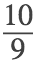Df(tm) % First Derivative Test yields absolute minimum since
ans = 0
% f'(t) < 0 for t < 10/9, f'(10/9) = 0, f'(t) > 0 for t > 10/9.
d_chk = sqrt(f(tm)) % Answer agrees with that obtained via vector methods.
d_chk =#### 9. [833/74]

The distance between parallel planes is computed using the formula given in 833/75, q.v.
syms x y z
plane1 = x - 2*y + 3*z + 0 == 0
plane1 =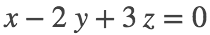plane2 = x - 2*y + 3*z - 1/3 == 0
plane2 =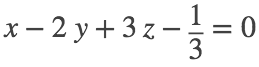d = abs(sym(0 + 1/3)) / sqrt(sym(1 + 4 + 9))
d =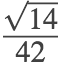d_appx = double(d) % in cm

#### 10. [833/80]

The distance between skew lines is computed using a scalar projection.
syms s t x y z
A = sym([1 2 6]), B = sym([2 4 8]) % A is a point on the first line
A =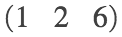B =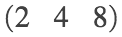v = B-A % direction vector for first line
v =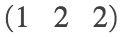P1 = x - y + 2*z + 1 == 0
P1 =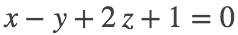P = sym([3 2 -1]), Q = sym([0 0 1]), R = sym([1 2 1])
P =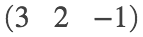Q =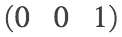R =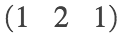PQ = Q-P, PR = R-P
PQ =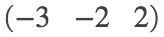PR =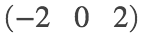c = cross(PQ, PR), n2 = -c/2
c =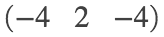n2 =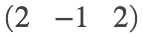P2 = dot(n2,[x y z]) == dot(n2,P)
P2 =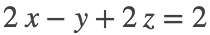l2 = solve([P1 P2], [x y z], 'ReturnConditions', true);
l2.x, l2.y, l2.z
ans = 3
ans =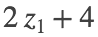ans =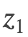L2 = [3 2*t+4 t]
L2 =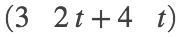w = diff(L2,t) % direction vector for second line
w =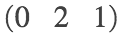c = cross(v,w)
c =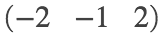S = subs(L2, t, 0) % point on second line
S =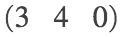u = S - A % vector connecting points on the two lines
u =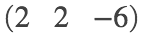d = abs(dot(c,u)) / norm(c) % distance between the skew lines: abs val of scalar projection
d = 6